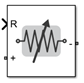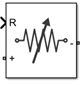# Variable Resistor

Model variable resistor

• Library:
• RF Blockset / Circuit Envelope / Elements

•## Description

The Variable Resistor block controls the output of RF Blockset™ feedback circuits using Simulink® controlled resistance in ohms. The minimum value of the resistance (Rmin) is an RF Blockset defined constant independent of the Simulink control signal. The block has two electrical terminals. One terminal is for the Simulink control signal and one terminal is for the RF Blockset signal.

Variable Resistor block mask icons are dynamic and show the current state of the applied noise parameter. This table shows you how the icons on this block vary based on the state of the Simulate noise parameter on the block.

Simulate noise: `on`Simulate noise: `off`## Ports

### Input

expand all

Simulink control signal, specified as a scalar.

Data Types: `double`

## Parameters

expand all

Select this parameter, to simulate thermal noise in the variable resistor. Then, in the Configuration block dialog box, also select the Simulate noise check box. By default, both Simulate noise check boxes are selected.

This parameter inserts a current noise source with the single-sided power density of 4 kT/R A2/Hz, where:

• k is the Boltzmann constant

• T is the value of the Temperature parameter, in degrees Kelvin. (Also located in the Configuration block.)

## Version History

Introduced in R2017b

expand all

Behavior changed in R2021b